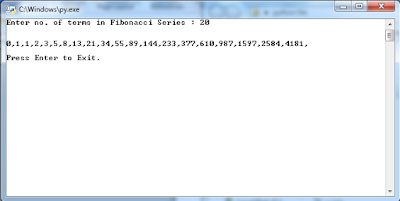## Tuesday, 29 May 2018

### To print ‘n terms of Fibonacci series using iteration using python programming.

``` n = int(input("Enter no. of terms in Fibonacci Series : ")) fTerm = 0 nTerm = 1 print("\n") for i in range(n):     if i<2:         print(i,end=",")     else:         temp = nTerm         nTerm = fTerm + nTerm         print(nTerm,end=",")         fTerm = temp input("\n\nPress Enter to Exit.") ```